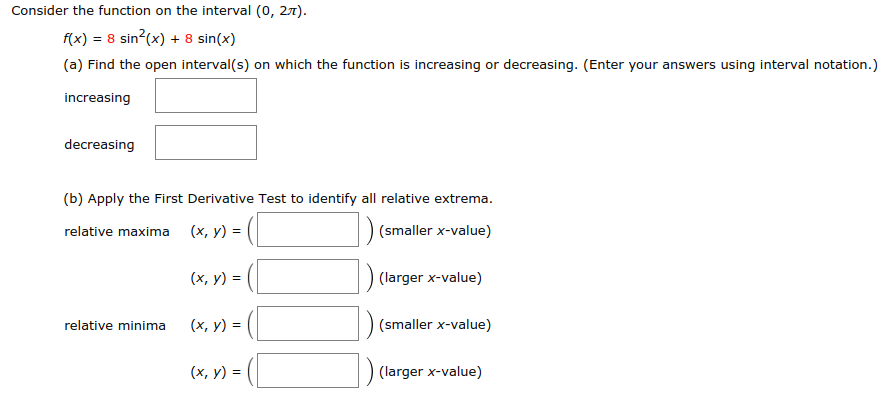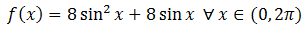# Consider the function on the interval (0, 27).x)8 sin(x) 8 sin(x)(a) Find the open interval(s) on which the function is increasing or decreasing. (Enter your answers using interval notation.)increasingdecreasing(b) Apply the First Derivative Test to identify all relative extrema(x, y) =(smaller x-value)(larger x-value)(smaller x-value)(largerx-value)relative maximarelative minima (x, y) -(x, y)

Question
64 viewshelp_outlineImage TranscriptioncloseConsider the function on the interval (0, 27). x)8 sin(x) 8 sin(x) (a) Find the open interval(s) on which the function is increasing or decreasing. (Enter your answers using interval notation.) increasing decreasing (b) Apply the First Derivative Test to identify all relative extrema (x, y) = (smaller x-value) (larger x-value) (smaller x-value) (largerx-value) relative maxima relative minima (x, y) - (x, y) fullscreen
check_circle

Step 1

Given function isStep 2

### Want to see the full answer?

See Solution

#### Want to see this answer and more?

Solutions are written by subject experts who are available 24/7. Questions are typically answered within 1 hour.*

See Solution
*Response times may vary by subject and question.
Tagged in

### Functions